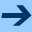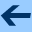Subtyping Rules

# Subtyping Rules

This section defines the subtyping rules for GOO in terms of subtype methods.

```(dm subtype? (t1|<union> t2|<type> => <log>)
(all? (op subtype? _ t2) (type-elts t1)))
(dm subtype? (t1|<type> t2|<union> => <log>)
(any? (op subtype? t1 _)) (type-elts t2)))
(dm subtype? (t1|<union> t2|<union> => <log>)
(all? (op subtype? _ t2)) (type-elts t1)))
(dm subtype? (t1|<class> t2|<class> => <log>)
(subclass? t1 t2))
(dm subtype? (t1|<singleton> t2|<class> => <log>)
(isa? (type-object t1) t2))
(dm subtype? (t1|<subclass> t2|<class> => <log>)
(subclass? <class> t2))
(dm subtype? (t1|<class> t2|<singleton> => <log>) #f)
(dm subtype? (t1|<singleton> t2|<singleton> => <log>)
(== (type-object t1) t2))
(dm subtype? (t1|<subclass> t2|<singleton> => <log>) #f)
(dm subtype? (t1|<class> t2|<subclass> => <log>)
(and (== t1 <class>) (== (type-class t2) <class>)))
(dm subtype? (t1|<subclass> t2|<subclass> => <log>)
(subclass? (type-class t1) (type-class t2)))
(dm subtype? (t1|<singleton> t2|<subclass> => <log>)
(and (isa? (type-object t1) <class>)
(subclass? (type-object t1) (type-class t2))))
(dm subtype? (t1|<product> t2|<type> => <log>) #f)
(dm subtype? (t1|<type> t2|<product> => <log>) #f)
(dm subtype? (t1|<product> t2|<product> => <log>)
(and (== (len (type-elts t1)) (len (type-elts t2)))
(all? (zipped subtype?) (zip (type-elts t1) (type-elts t2)))))
(dm subtype? (t1|<product> t2|<class> => <log>)
(subtype? <tup> t2))
```Subtyping Rules